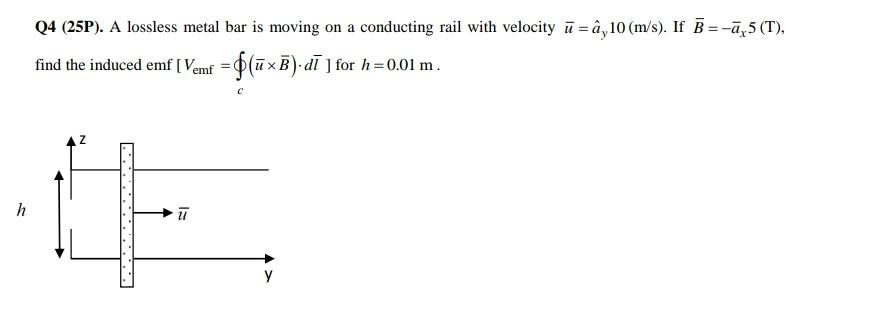Home / Expert Answers / Electrical Engineering / nbsp-q4-25p-a-lossless-metal-bar-is-moving-on-a-conducting-rail-with-velocity-bar-u-ha-pa313

# (Solved):   Q4 (25P). A lossless metal bar is moving on a conducting rail with velocity $$\bar{u}=\ha ...Q4 (25P). A lossless metal bar is moving on a conducting rail with velocity \( \bar{u}=\hat{a}_{y} 10(\mathrm{~m} / \mathrm{s})$$. If $$\bar{B}=-\bar{a}_{x} 5$$ (T), find the induced emf $$\left[V_{\mathrm{emf}}=\oint_{c}(\bar{u} \times \bar{B}) \cdot d \bar{l}\right]$$ for $$h=0.01 \mathrm{~m}$$.

We have an Answer from Expert